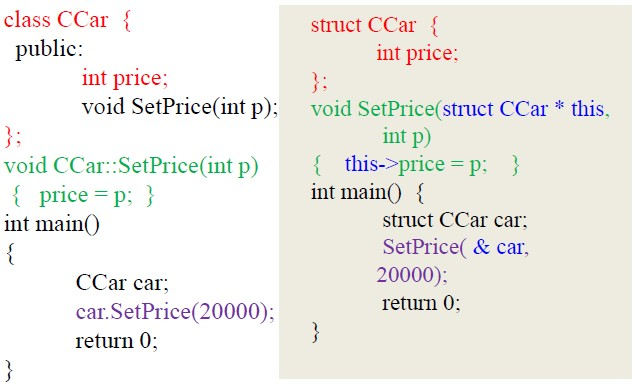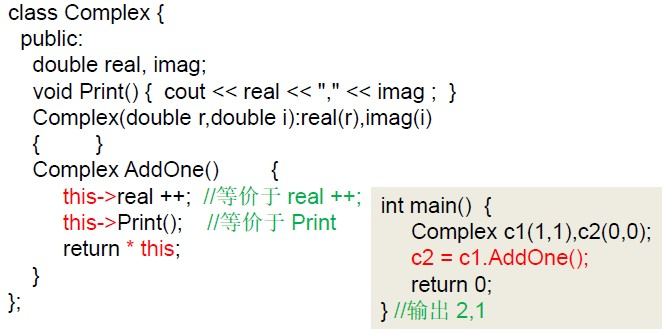#C++面向对象程序设计课程笔记（第三周）

0
0
01. 云栖社区>
2. 博客>
3. 正文

## 第一节 this指针

1.C++程序到C程序的翻译C++中的class在C语言中并不存在，因此可以用struct（结构体）来代替，成员变量也可以直接用域来代替，但是类中的成员函数却无法在C语言中直接表示，所以只能把SetPrice设置为全局函数。由于是全局函数，为了能让其使用结构体内的数据，故在SetPrice中增加了一个参数struct CCar *this，这样就可以在函数体中把p的值传递给结构体中的price，实现了跟成员函数一样的功能，同理，在main()中SetPrice函数执行也得把car的地址传递过去。

2.this指针作用

（1）非静态成员函数中可以直接使用this来代表指向该函数作用的对象的指针。``````class A

{

int i;

public:

void Hello()

{cout<<“”Hello”<<endl;}

};

int main()

{

A *p=NULL;

p->Hello();

}``````

void Hello(A *this){cout <<”Hello”<<endl;

## 第二节 静态成员变量

1.静态成员基本概念

sizeof运算符在计算类的大小的时候不会计算静态成员变量。

2.如何访问静态成员

（1）类名::成员名

（2）对象名.成员名

（3）指针->成员名

CRectangle *p=&r;p->PrintTotal();

（4）引用.成员名

CRectangle &ref =r; int n = ref.nTotalNumber;

``````CRectangle::CRectangle(int w_,h_) {

w = w_;

h = h_;

nTotalNumber++;

nTotalArea += w* h;

}

CRectangle::~CRectangle(){

nTotalNumber–;

nTotalArea -= w*h;

}

void CRectangle::PrintTotal(){

cout<<nTotalNumber<<“,”<<nTotalArea<<endl;

}``````

``````CRectangle::CRectangle(CRectangle &r)

{

w = r.w;h=r.h;

nTotalNumber ++;

nTotalArea += w*h;

}``````

## 第三节 成员对象和封闭类

1.基本概念

``````class CTyre

{

private:

int width;

public:

};

class CEngine{};

class CCar{//封闭类

private:

int price;

Ctyre tyre;//成员对象

CEngine engine;//成员对象

public:

CCar(int p, int tr,int tw);

};

CCar::CCar(int p,int tr,int w):price(p),tyre(tr,w)//engine使用无参构造函数构造

{};

int main()

{

CCar car(20000,17,225);

return 0;

}``````

2.封闭类构造函数和析构函数的执行顺序

（1）封闭类对象生成时，先执行所有对象成员的构造函数，然后才执行封闭类的构造函数；

（2）对象成员的构造函数调用次序和对象成员在类中的说明次序一致，与它们在成员初始化列表中出现的次序无关；

（3）当封闭类的对象消亡时，先执行封闭类的析构函数，然后再执行成员对象的析构函数。次序和构造函数的调用次序相反。

3.封闭类的复制构造函数

``````Class A

{

public:

A(){cout<<“default”<<endl;}

A(A&a){cout<<“copy”<<endl;}

};

class B{A a;};

int main()

{

B b1,b2(b1);

return 0;

}``````

## 第四节 友元（friends）

1.友元函数

``````Class B{

public:

void function();

};

Class A{

friend void B::function();

};``````

2.友元类

``````Class CCar

{

private:

int price;

friend class CDriver;//声明CDriver为友元类

};

class CDriver

{

public:

CCar myCar;

void ModifyCar(){

myCar.price += 1000;

//因为CDriver是CCar的友元类，故可以访问其私有成员

}

};

int main(){return 0;}``````

## 第三节 常量对象、常量成员函数和常引用

1.常量成员函数

``````class Sample{

private:

int value;

public:

Sample(){}

void SetValue(){}

};

const Sample Obj;//常量对象

Obj.SetValue();//错误。常量对象只能使用构造函数、析构函数和右const说明的函数（const方法）``````

``````class Sample{

public:

int value;

void func(){};

Sample(){}

void GetValue() const;

};

void Sample::GetValue() const{

value = 0;//wrong

func();//wrong

}

int main(){

const Sample o;

o.value = 100;//err常量对象不可被修改

o.func();//常量对象上面不能执行非常量成员函数

o.GetValue();//OK常量对象可以执行常量成员函数

return 0;

}``````

2.常量成员函数的重载

3.常引用

``````class Sample{

};

void PrintfObj(Sample & o){

}``````

``````class Sample{

};

void PrintfObj(const Sample & o){

}``````

+ 关注